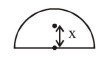# The centre of mass of a solid hemisphere of radius

Question:

The centre of mass of a solid hemisphere of radius $8 \mathrm{~cm}$ is $X \mathrm{~cm}$ from the centre of the flat surface. Then value of $x$ is

Solution:

$x=\frac{3 R}{8}=3 \mathrm{~cm}$

$x=3$